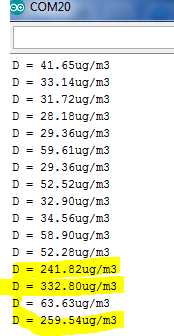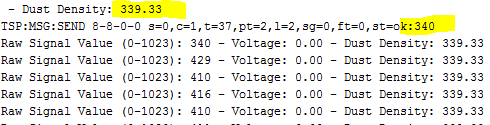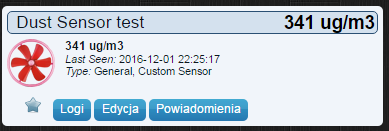# Dust sensor and wrong values

• Re: Dust Sensor

My Sensors team,

I'd like to refresh this topic with a question.
I'm trying to setup dust senor by my own on MySensors 2.0 lib., but have dificulities with correct GP2Y1010AU0F values in my controller (Domoticz).

To check if my wiring is ok I've used regular arduino sketch:

``````#define MIN_VOLTAGE     600 // mv - the lower threshold voltage range for the lack of dust
#define VREF           1100 // mv - reference voltage of the comparator
#define PIN_LED           3 // pin number ILED
#define PIN_ANALOG        1 // pin number AOUT
#define MAX_ITERS        10 // liczba pomiarow do sredniej

int ADC_VALUE; // read value A1
int ITER; // value read
float VOLTAGE; // voltage value
float DUST; // result
float AVG_DUST; // average result

void setup(void)
{
analogReference(INTERNAL);

Serial.begin(9600);

pinMode(PIN_LED, OUTPUT);
digitalWrite(PIN_LED, LOW);
}

float computeDust()
{
// Flash IR, wait 280ms, read ADC voltage
digitalWrite(PIN_LED, HIGH);
delayMicroseconds(280);
ADC_VALUE = analogRead(PIN_ANALOG);
digitalWrite(PIN_LED, LOW);

// Calculate to mV. whole multiplied by 11, because in the module
// applied voltage divider 1k/10k
VOLTAGE = (VREF / 1024.0) * ADC_VALUE * 11;

// Calculate pollution if the measured voltage over prog

if (VOLTAGE > MIN_VOLTAGE)
{
return (VOLTAGE - MIN_VOLTAGE) * 0.2;
}

return 0;
}

void loop(void)
{
AVG_DUST = 0;
ITER = 0;

while (ITER < MAX_ITERS)
{
DUST = computeDust();
// For medium liczmy only correct measurements
if (DUST > 0)
{
AVG_DUST += DUST;
ITER++;
delay(50);
}
}

AVG_DUST /= MAX_ITERS;

Serial.print("D = ");
Serial.print(AVG_DUST);
Serial.println("ug/m3");

delay(500);
}

``````

The output seems to be ok:(yellow marked is smoke test).

Then I've applied following sketch:

``````// Enable debug prints
#define MY_DEBUG

// Enable and select radio type attached
#define MY_RADIO_NRF24
//#define MY_RADIO_RFM69

#include <SPI.h>
#include <MySensors.h>

#define CHILD_ID_DUST 0
#define DUST_SENSOR_ANALOG_PIN 1
#define DUST_SENSOR_DIGITAL_PIN 3

const int numreadings = 60;

unsigned long SLEEP_TIME = 1000;

int val = 0;
float valDUST =0.0;
float lastDUST =0.0;
int samplingTime = 280;
int deltaTime = 40;
int sleepTime = 9680;
float voMeasured = 0;
float calcVoltage = 0;
float dustDensity = 0;

MyMessage dustMsg(CHILD_ID_DUST, V_LEVEL);

void setup(){
pinMode(DUST_SENSOR_DIGITAL_PIN,OUTPUT);
}

void presentation() {
// Send the sketch version information to the gateway and Controller
sendSketchInfo("Dust Sensor", "1.0");

// Register all sensors to gateway (they will be created as child devices)
present(CHILD_ID_DUST, S_DUST);
}

void loop() {
float cumsum = 0;
float temp = 0;
// float temp1 = 0;
// float cum_density = 0;
for (int sample = 0; sample < numreadings; sample ++){
digitalWrite(DUST_SENSOR_DIGITAL_PIN,LOW); // power off the LED
delayMicroseconds(samplingTime);
uint16_t voMeasured = analogRead(DUST_SENSOR_ANALOG_PIN);// Get DUST value
delayMicroseconds(deltaTime);
digitalWrite(DUST_SENSOR_DIGITAL_PIN,HIGH); // turn on the LED
// 0 - 5V mapped to 0 - 1023 integer values
// recover voltage
temp = voMeasured * (5.0 / 1024.0);
cumsum = cumsum + temp;// cumulative sum over 60 seconds
delay(500);
Serial.print("Raw Signal Value (0-1023): ");
Serial.print(voMeasured);
Serial.print(" - Voltage: ");
Serial.print(calcVoltage);
Serial.print(" - Dust Density: ");
Serial.println(dustDensity);
}

// linear eqaution taken from http://www.howmuchsnow.com/arduino/airquality/
// Chris Nafis (c) 2012
dustDensity = (0.17 * cumsum - 0.1)* (1000/60);

Serial.print(" - Dust Density: ");
Serial.println(dustDensity); // unit: ug/m3

send(dustMsg.set((int)ceil(dustDensity)));

sleep(SLEEP_TIME);
}
``````

and the output is:In Domoticz it looks:It seems that my room air polution is super high :), but, it isn'tIdeas, what should be changed in the code?

Best,

• @1kohm how come you don't use the computedust function in the second sketch?

• Hi,

becouse I don't konw it and where to put itAdvise would be apriciate.

best,

• OK,
I was tired...
I've figure it out and have sketch runningBeta version:

``````// Enable debug prints
#define MY_DEBUG

// Enable and select radio type attached
#define MY_RADIO_NRF24
//#define MY_RADIO_RFM69

#include <SPI.h>
#include <MySensors.h>

#define CHILD_ID_DUST 0
#define MIN_VOLTAGE              600 // mv - the lower threshold voltage range for the lack of dust
#define VREF                    1100 // mv - reference voltage of the comparator
#define DUST_SENSOR_DIGITAL_PIN    3 // pin number ILED
#define DUST_SENSOR_ANALOG_PIN     1 // pin number AOUT
#define MAX_ITERS                 10 // liczba pomiarow do sredniej

unsigned long SLEEP_TIME = 1000;    // Sleep time between reads (in milliseconds)

int SAMPLING_TIME = 280;
int ADC_VALUE; // read value A1
int ITER; // value read
float VOLTAGE; // voltage value
float DUST; // result
float AVG_DUST; // average result

MyMessage dustMsg(CHILD_ID_DUST, V_LEVEL);

void setup(){
analogReference(INTERNAL);
Serial.begin(115200);
pinMode(DUST_SENSOR_DIGITAL_PIN,OUTPUT);
digitalWrite(DUST_SENSOR_DIGITAL_PIN, LOW);
}

void presentation() {
// Send the sketch version information to the gateway and Controller
sendSketchInfo("Dust Sensor", "1.0");

// Register all sensors to gateway (they will be created as child devices)
present(CHILD_ID_DUST, S_DUST);
}

float computeDust()
{
// Flash IR, wait 280ms, read ADC voltage
digitalWrite(DUST_SENSOR_DIGITAL_PIN, HIGH);
delayMicroseconds(SAMPLING_TIME);
ADC_VALUE = analogRead(DUST_SENSOR_ANALOG_PIN);
digitalWrite(DUST_SENSOR_DIGITAL_PIN, LOW);

// Calculate to mV. whole multiplied by 11, because in the module
// applied voltage divider 1k/10k
VOLTAGE = (VREF / 1024.0) * ADC_VALUE * 11;

// Calculate pollution if the measured voltage over prog

if (VOLTAGE > MIN_VOLTAGE)
{
return (VOLTAGE - MIN_VOLTAGE) * 0.2;
}

return 0;
}

void loop()
{
AVG_DUST = 0;
ITER = 0;

while (ITER < MAX_ITERS)
{
DUST = computeDust();
// For medium liczmy only correct measurements
if (DUST > 0)
{
AVG_DUST += DUST;
ITER++;
delay(50);
}
}

AVG_DUST /= MAX_ITERS;

Serial.print("D = ");
Serial.print(AVG_DUST);
Serial.println("ug/m3");

send(dustMsg.set((int)ceil(AVG_DUST)));

sleep(SLEEP_TIME);

}
``````

Values are send, but I want to send to gateway values exact as computed by sketch, wih 4 ditits - D = 60.79ug/m3

But sketch is sending only 2 digit - ok:61

``````D = 60.79ug/m3
TSP:MSG:SEND 8-8-0-0 s=0,c=1,t=37,pt=2,l=2,sg=0,ft=0,st=ok:61
D = 59.61ug/m3
TSP:MSG:SEND 8-8-0-0 s=0,c=1,t=37,pt=2,l=2,sg=0,ft=0,st=ok:60
``````

I guess that I should change something here:

``````  send(dustMsg.set((int)ceil(AVG_DUST)));
``````

But not know what to change.
Help here.

• @1kohm Great work! I think this is what you want:

``````send(dustMsg.set(AVG_DUST,2));
``````

The number 2 means two decimals

• @mfalkvidd, Thanks!

Works like a charm now!

I've included now DHT sensor in this sketch as wellTesting so far.
So, another questionTo tell sketch when to send data I'm using:

``````unsigned long SLEEP_TIME = 1000;
``````

Now it's sending every 1 second. But my goal is to change it to send data only when there's a value change.

I've found following code (it's for Dallas temp sensor), that is sending only changed values:

``````void loop()
{
// Fetch temperatures from Dallas sensors
sensors.requestTemperatures();

// query conversion time and sleep until conversion completed
int16_t conversionTime = sensors.millisToWaitForConversion(sensors.getResolution());
// sleep() call can be replaced by wait() call if node need to process incoming messages (or if node is repeater)
sleep(conversionTime);

// Read temperatures and send them to controller
for (int i=0; i<numSensors && i<MAX_ATTACHED_DS18B20; i++) {

// Fetch and round temperature to one decimal
float temperature = static_cast<float>(static_cast<int>((getConfig().isMetric?sensors.getTempCByIndex(i):sensors.getTempFByIndex(i)) * 10.)) / 10.;

// Only send data if temperature has changed and no error
#if COMPARE_TEMP == 1
if (lastTemperature[i] != temperature && temperature != -127.00 && temperature != 85.00) {
#else
if (temperature != -127.00 && temperature != 85.00) {
#endif

// Send in the new temperature
send(msg.setSensor(i).set(temperature,1));
// Save new temperatures for next compare
lastTemperature[i]=temperature;
}
``````

How to implement it in my case?
Help here would be great

Best,